# Another question to prove you're smart!

1. Okay you wise guys/gals,

You have 80 potatoes. You take the potatoes and divide them by half then add 6, multiplied by 5. How many potatoes do you have?

1. According to your answer there are 830 potatoe pieces but actually you have 80 whole potatoes. Therefore the correct answer is 80 whole potatoes.

2. What Kind of Potatoes?

1. They are small with cute little ears ROFL.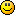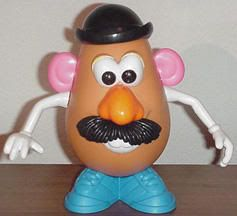1. SO COOL!
The answer to the question is simple!
The Bus driver did it!
Pass to the left!

1. Yes let's blame the bus driver haha.
He did it!!!!!!
Mr Potato head was his accomplice in crime.

2. 40

Divide them by half, half of 80 is 40

80/40=2

=8

multiply by 5

=40

or 2 plus 6 multiplied by 5=32, but you put a comma which would infer the order of operations above.

3. I am not. In your sense.4. I would just divide the potatoes, drizzle them with olive oil, then roast them and multiply the deliciousness with rosemary.

1. LMAO and sit on the sofa with my feet on a poofa, and watch Masterchef on telly while indulging.

5. If you divide them by half (i.e. cut them in two) you'd have 160 potato pieces. If you add 30 more potatoes, you'd have 160 potato pieces and 30 potatoes.

6.0
ralwusposted 13 years ago

230

7. Or, if you want to follow the operations chronologically, 830.

I don't really like the wording in that though, I think it's more appropriate to add 30 (add 6, multiplied by 5)

1. You're being too literal!

It's not in the wording..

Thiiiiiiiink....

Still thinking?

1. I usually am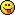What was the answer though, out of curiousity?

Start again.
80 potatoes. Half them (as we do, becaue a whole potato unless they are small takes too long to cook) makes 160. Add 6. OK That's 166. Then multiply by 5 = 830 (actually I thought it was 560 the first time - it's late OK my head isn't so good).
But again, it could be a trick question.
The line asked "divide them by half" which isn't necessarily halfing them.

9. Duh! You have 80 potatoes. You can't have any more or less thanyou started off with!

1. Izzy, if you still have that match from Waffle's other thread you could smoke the 80 potatoes.10. 80 divided by half (half of 80 is 40 (40/80=2)) add 6 (2+6=8) multiplied by 5 (8x5=40)
40 is what I come up with.

1. At least I won't go down in flames all alone. Cumonnnnnn 40!

2. 40/80 = . 5 or 1/211. hmmm...

70...1. Did you eat 10??? lol

1. nope... I did this...(80 potatoes/2) = 40
(6, multiplied by 5) = 30
equals...
...
wait for it
...
701. Right there are several ways the actual sum can be looked at.
The rest of the question only mentioned sums, nothing to do with your 80 potatoes.
So you still have 80 potatoes?
I'm getting a headache, I think I'll go to bed...

12. 70

EDIT: LOL. we cross posted(80/2)+(5x6)=70

1. you just copied my answer... haha1. LOL I swear I hit reply, then got distracted for a mo - and when I posted, yours was there already...

1.13. Come on, WaffleCheese...

Give us the right answer...14.0
ralwusposted 13 years ago

i think he is busy getting another ready

The original question:
[You have 80 potatoes. You take the potatoes and divide them by half then add 6, multiplied by 5. How many potatoes do you have?]

If you divide the 80 potatoes 'by' half, you'd have 40 potatoes.  If you divided the potatoes 'in' half, you'd have 160 potato halves, but still no more than 80 potatoes.  I'm assuming 40.

Ok..I think the rest of the question after the 'divide them by half' is a deception.

The real answer is in fact be 40 potatoes.

Ok. WaffleCheese quit waffling...is it in fact 40?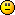1. What's happened to the math rules that we've all learned in school?

http://www.purplemath.com/modules/numbprop.htm

Since when does one not multiply/divide, prior to doing addition/subtraction?16. Excuse-moi? Didn't I say that about 15 million posts back? AND I wasn't the first...Pretty Dark Horse said it too..then you said to look at the problem in a simpler fashion or words to that effect.
So I went back to the 80 tatties you started with..
Right I really do have a headache now...I am going!

17. Haha..

Well, I must apologize.

I am at work right now (don't tell my boss) and so I'm unable to read each post.

Izzy, if it's any consolation, you're brilliant!

Wafflecheese

1. Lay another one on us Sam I am ready.18. 830 huh?  Well yes, but only if you consider the original 80 potatoes were cut 'in half', and not 'by half' as you put it. At that point there would still be 80 potatoes.  Your phrasing is faulty if your intended answer was 830.  830 what?

You also didn't specify whether to add 6 'potatoes', or just the number 6.

I still think the final adding and multiplying are just window-dressing.

The last part of the question is the most telling:
"How many potatoes do you have?"

No..I'm sorry, the answer is stil 40 (or 80) potatoes.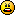So there.  Good night.

19.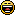20. Complete the last two in this sequence: 1=3, 2=3, 3=5, 4=4, 5=4, 6=3, 7=5, 8=5, 9=4, 10=3, 11=?, 12=?

1. Won't be a tic Waffle I am in the process of studying this one while trying to peel some prawns for lunch.1. 11= 6   12= 6. Is that right? Oops spilled a prawn shell down my shirt LMAO.

2. 11 = 6, 12 = six. as BP said. or to put it another way eleven has 6 letters and twelve has six letters, that's the sequence your using.
The other problem is solved by the mathematical rule we used in school of BODMAS the order of calculations in an equation;
Brackets, Of, Division, Multiplication, Addition, Subtraction.

Good puzzles though. Got any more?

21. Waffle where are you?
Quick have a coffee break when no-one is looking at work to tell me if I am right or wrong. That took hard work lol eating and thinking at the same time.

1. Woo hoo Waffle that has just waffled my socks.
See everyone the Blonde did it. I will gladly do autographs later LMAO.

22. Sorry, just got home from work!

haha

23. Actually, this is a tricky question. I used to have a friend, a math teacher and he was always challenging my son with these kind of questions. By coincidence, he gave him this one and I remember the solution.  They're hoping that you do this:

80/2 + 6 x 5.

What you should actually do is this:

80 divided by 1/2 + (6 x 5).

80 divided by 1/2 is the same as 80 x 2 (by the invert-and-multiply rule), so 80 divided by half is actually 160.

So now you have 160 + (6 x 5).

The order of operations dictates that you multiply before you add. 6 x 5 is 30. 160 plus 30 is 190.

The correct answer is 190. The answer most people would get is 230 (the result of 40 + 6 x 5).

24. With all of the dividing I would probably nibble on 1/25 and then I would be to exhausted to count.1. I can lend you one of my poofas AE to rest your feet on while you think lol.1. Heheheheheh! Please me toesy and feetsy need a little rest!1. A little comfy poofa for poor worn out AE coming up.25. The number sequence that blondepoet got right (congrats, BTW!) - that's just another version of "The Magic Number."  Four is The Magic Number, because all numbers eventually lead back to four by this process.

In English, that is.  (Oops, did I give it away?)26. Mr,Tomato your question is too complicated. Let me think about it again I will get across to you very soon

working